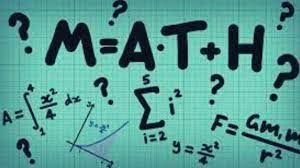# Math riddles with answers: Math is a tough subject. Are you good at it? Let's check it!

Math is a tough subject. Math is an easy subject, said no man ever. Are you confident about your mathematical skills? Let's check it.Talk about language or science scores among a group of people and they may show up as confident people, but the moment you discuss math marks, you'll see people hiding their faces.

Since childhood, there is a conditioned fear among all of us that math is a tough subject. Well, the fear is not for nothing. Math is indeed a tough subject.

The subject has been blamed for shaking both academic records and confidence.

Today, we plan to act like Satan and shake your confidence again, with these math riddles.

## Math Riddle 1:

What comes in the empty space?

1,8,27,64,?,216

## Math Riddle 2:

What comes in the empty space?

A, Z, D, Y, G, X,?,?

## Math Riddle 3:

What comes in the empty space?

CD, HI, MN,?

## Math Riddle 4:

The product of 2 numbers is 1575 and their quotient is 9/7. Then the sum of the numbers is:

## Math Riddle 5:

The sum of the squares of two natural consecutive odd numbers is 394. The sum of the numbers is?

Math Riddle 1:

What comes in the empty space?

1,8,27,64,?,216

125

Math Riddle 2:

What comes in the empty space?

A, Z, D, Y, G, X,?,?

J, W

Math Riddle 3:

CD, HI, MN,?

RS

Math Riddle 4:

The product of 2 numbers is 1575 and their quotient is 9/7. Then the sum of the numbers is?

Let's assume the numbers to be x and y.

We know xy = 1575

And x/y = 9/7

We know xy/x/y = 1575/9/7

We know y2 = 1225

We know y = 35 and x = 45

We know The sum of the numbers = 45+35

= 80

Math Riddle 5:

The sum of the squares of two natural consecutive odd numbers is 394. The sum of the numbers is?

Let's assume the consecutive odd numbers to be x and (x+2)

We know that x2 + (x + 2)2 = 394

We know x2 + x2 + 4x + 4 = 394

We know 2 x2 +4x – 390 = 0

We know x2 + 2x – 195 = 0

We know (x +15) (x-13) = 0

We know x = 13

We know Required sum = 13 +15 =28

Did your confidence shake? Do you still consider yourself smart? Well, even if you couldn't solve these, you are still smarter than you think you are. Smartness is not measured by your skill to solve 5 riddles, but by your ability to walk through the riddles, fail multiple times, and then finally come out as a winner some time.

Optical Illusion: Can you find the hidden chick in this fruit market in just 5 seconds?

Get the latest General Knowledge and Current Affairs from all over India and world for all competitive exams.
खेलें हर किस्म के रोमांच से भरपूर गेम्स सिर्फ़ जागरण प्ले पर